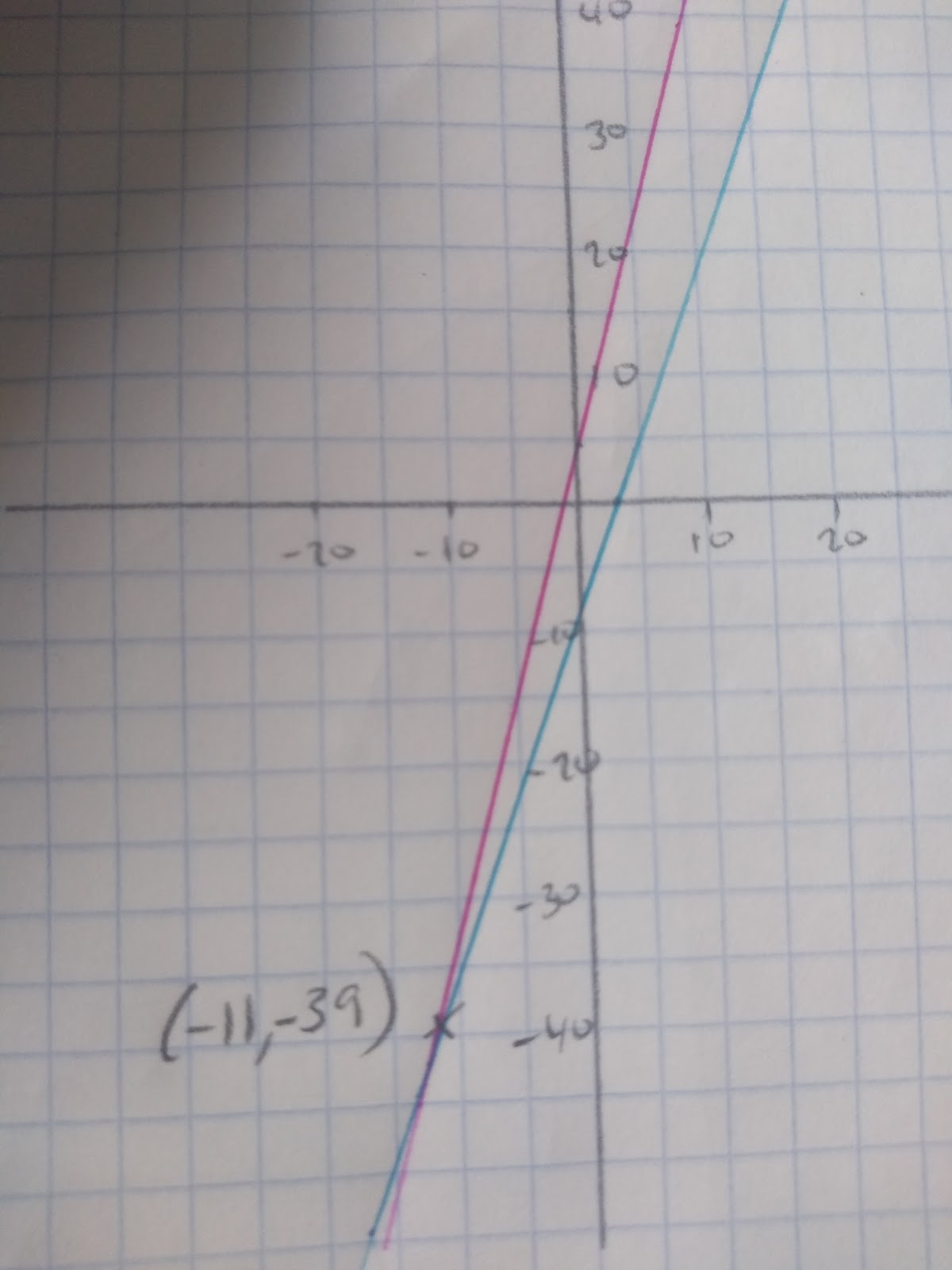# Solving Equations

## Solving linear equations

To solve equations we need to think about how terms are linked together. If we take a variable, let’s call it s and then decide to add 4 to s and then to multiply it by 3 and make it equal to 30 we have an equation:

s

s+4

3(s+4) = 30

To unravel this equation we need to do the reverse to both sides.

3(s+4) = 30 Instead of multiplying by 3 I will have to divide both sides by 3

s+4 = 10_ _Instead of adding four I will subtract 4

s = 6 Then I have my value of s!

The key thing to remember is that whatever you do to one side of the equals sign, you__ must__ do to the other!

## Solving linear equations with an unknown on both sides

You will also have to solve equations with unknowns on both side. For example:

4x + 6 = 3x - 7

To start, aim to get the same terms on the same sides. In this case I would take away 3x from both sides of the equation:

4x + 6 = 3x - 7

x + 6 = - 7

Then I would take away 6 from both sides:

x = -13

Check to make sure your answer is correct. On the higher paper you will be expected to solve more difficult equations, but the same process remains.

## Using graphs to solve equations

If you have two equations you can use a graph to solve them, to find which values of x and y fit both equations.

Solving Simultaneous Equations Graphically

For example, if we wanted to solve 4x + 5 = 3x - 6 we could simply draw the lines. y = 4x + 5 and y = 3x - 6 and our answer will be the point at which they intercept.As you can see from the graph, the point where the two lines cross is the solution to the simultaneous equation.

x = -11 and y = -39 The x and y values will be the same!

At this point

-39=4(-11)+5

-39=3(-11)-6

Solve this equation 4(7x + 3) = 40
1
8x -4 =3x +6
Your answer should include: 0.5 / 1/2